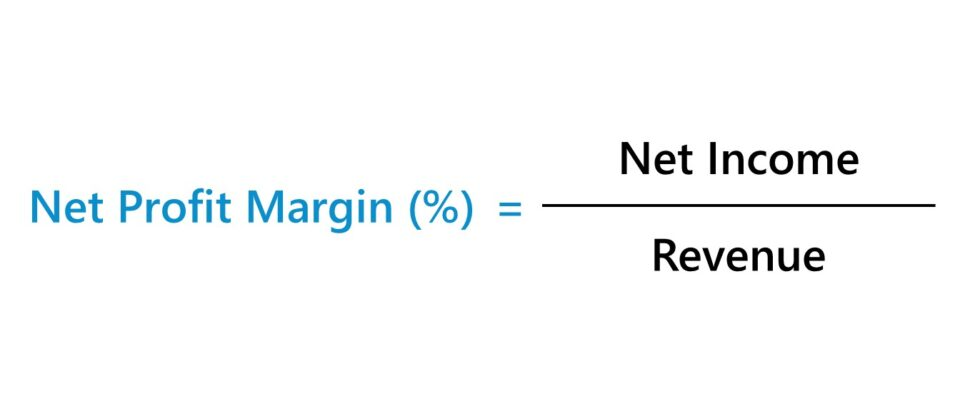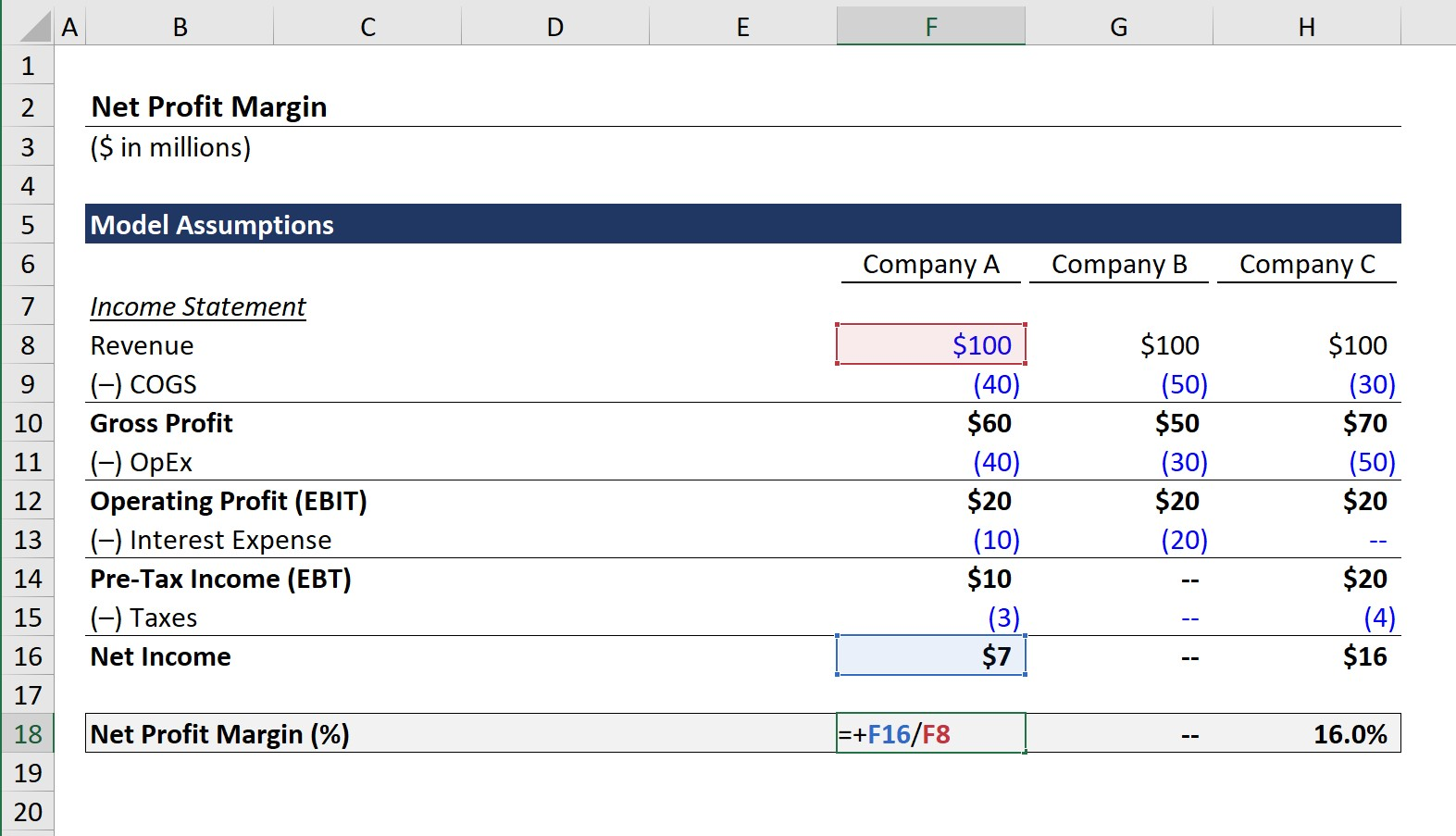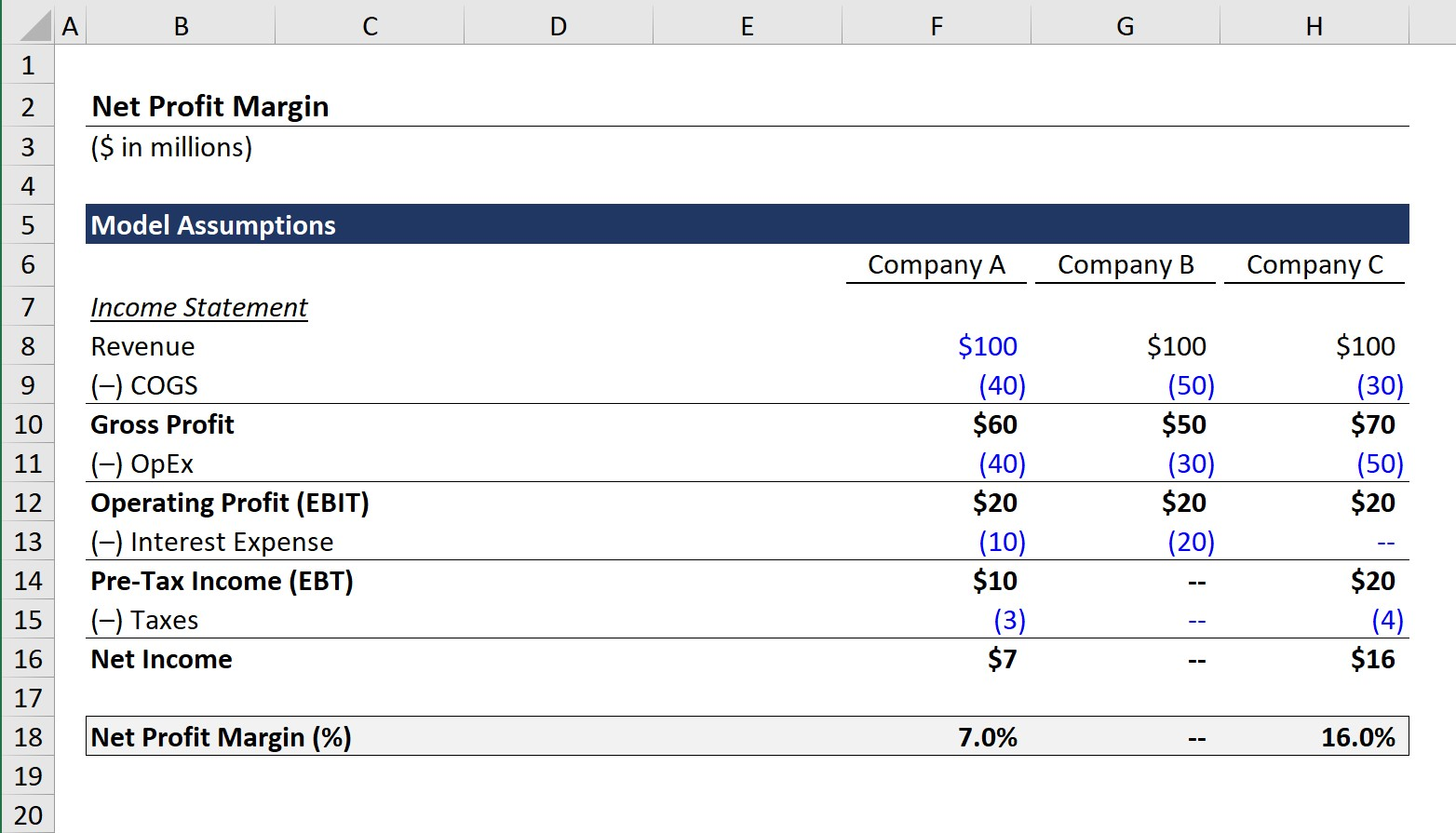# Net Profit Margin

Guide to Understanding the Net Profit Margin## How to Calculate Net Profit Margin (Step-by-Step)

The net profit margin, expressed as a percentage, answers the following question, “How much in profits is kept by the company for each dollar of revenue?”

For example, if a company has a net margin of 20%, \$0.20 in net income is generated for each \$1.00 of revenue.

The net margin calculation divides a company’s net income by the revenue in the corresponding period.

Both the revenue and net income of a company can be found on the company’s income statement, with the latter being calculated as revenue after subtracting all expenses.

Income Statement Line Items:

• Net Revenue
• Less: Cost of Goods Sold (COGS)
• Gross Profit = Revenue – COGS
• Less: Operating Expenses (OpEx)
• Operating Income (EBIT) = Gross Profit – OpEx
• Less: Non-Operating Expenses
• Pre-Tax Income (EBT) = EBIT – Non-Operating Expenses
• Less: Taxes
• Net Income = EBT –Taxes

## Net Profit Margin Formula

The net profit margin formula is as follows.

Net Profit Margin = Net Income ÷ Revenue
• Net Income → The “bottom line” of the income statement is net income, which measures a company’s post-tax earnings in a given period, once all operating and non-operating expenses have been deducted.
• Revenue → The “top line” of the income statement is revenue, which is the total monetary value of goods and/or services sold (and “earned” per accrual accounting standards) by a company over a given period.

To adjust the value into percentage form, you’d need to multiply the value by 100.

## What is a Good Net Profit Margin?

What a “good” net profit margin is will vary considerably by the industry, as well as the size and maturity of the companies under comparison.

As a general rule of thumb, net margins in excess of 10% are considered to be healthy, but the metric shouldn’t be taken at face value and requires more in-depth analysis, as our next section will explain.

Net income represents the accounting profits of a company, which means the metric is vulnerable to potential accounting manipulation and could show a misleading portrayal of profitability.

The concerns around the net margin metric stem from the shortcomings of accrual accounting, or more specifically, the potential for discretionary management decisions that could skew earnings.

## How to Interpret Net Profit Margin?

For example, a company’s net profit margin could be higher than its comparable peers due to the use of longer useful life assumptions for depreciating its fixed assets (PP&E), the lack of debt financing (i.e. resulting in lower or no interest expense), or operating in a state/country with more favorable tax structures.

Despite showing a higher net profit margin, the reality is that such differences are caused by non-operating factors, differences in accounting methods, and other discretionary decisions, rather than the company necessarily performing better than its peers.

The amount of debt on the balance sheet is one of the more significant considerations, as companies that opt to grow using debt financing rather than equity financing will incur higher interest expenses – which will effectively cause their net profit margin to decline.

Therefore, while higher net profit margins are typically perceived as positive signs, it is important to keep in mind the limitations around the net income metric and accrual accounting.

With that said, net income is a poor metric for comparability due to the aforementioned pitfalls, which directly impact the reliability of the net profit margin metric as a point of comparison.

## Net Profit Margin Calculator

We’ll now move to a modeling exercise, which you can access by filling out the form below.Submitting ...

## 1. Income Statement Assumptions

In the first section of our net profit margin modeling exercise, we’ll start with the income statement assumptions.

We’ll be comparing the net profit margin of three companies, with each having a different set of financial assumptions.

Income Statement – Company A

• Revenue: \$100m
• Cost of Goods Sold (COGS): –\$40m
• Operating Expenses (OpEx): –\$40m
• Interest Expense: –\$10m
• Tax Rate: 30%

Income Statement – Company B

• Revenue: \$100m
• Cost of Goods Sold (COGS): –\$50m
• Operating Expenses (OpEx): –\$30m
• Interest Expense: –\$20m
• Tax Rate: 25%

Income Statement – Company C

• Revenue: \$100m
• Cost of Goods Sold (COGS): –\$30m
• Operating Expenses (OpEx): –\$50m
• Interest Expense: \$0m
• Tax Rate: 20%

Note that the operating profit margin – i.e. EBIT divided by revenue – is 20% in all three cases.

Different approaches towards depreciation of PP&E and other accounting decisions such as inventory recognition (i.e. LIFO vs. FIFO) would be embedded within the COGS or OpEx line items.

However, the major differences in our example do not begin to emerge until the interest expense line item, which is just one example of a non-operating expense.

• Interest Expense, Company A = \$10m
• Interest Expense, Company B = \$20m
• Interest Expense, Company C = \$0

The more debt that a company holds on its balance sheet, the greater its interest expense will be – all else being equal.

In addition, the tax rate differs for each company, with Company A having the highest tax rate and Company C having the lowest tax rate.

## 2. Net Profit Margin Calculation Example

Once the stated assumptions have been entered, we are able to calculate the net income for each individual company:

• Net Income, Company A = \$7m
• Net Income, Company B = \$0m
• Net Income, Company C = \$16m

Company C has the highest net income out of the three, with Company B at a break-even point (i.e. revenue = total expenses).

If we divide each net income figure by the revenue amount, we arrive at the net profit margin for all three companies.For instance, if we divide Company A’s net income by its revenue, we get the following:

• Net Profit Margin = \$7m ÷ \$100m = .07, or 7.0%

## 3. Net Profit Margin Ratio Analysis

The calculated net profit margins for each company are listed below.

• Company A – Net Profit Margin: 7.0%
• Company B – Net Profit Margin: 0.0%
• Company C – Net Profit Margin: 16.0%

If interpreted at face value, we’d say that Company C is the most efficient at running its operations and turning revenue into net income.

However, as opposed to being a sole function of operating profitability and efficiency at utilizing its assets, the differences between each company are in fact attributable to the inconsistencies in debt and tax jurisdictions.

This exercise demonstrates that management-specific accounting policies can misconstrue margins. Further diligence into each company’s financials would be required to make a true determination around which company is the best operated.Step-by-Step Online Course

### Everything You Need To Master Financial Modeling

Enroll in The Premium Package: Learn Financial Statement Modeling, DCF, M&A, LBO and Comps. The same training program used at top investment banks.Inline Feedbackscalcuing
February 7, 2022 12:36 am

Both the revenue and net income of a company can be found on the company’s income statement, with the latter being calculated as revenue after subtracting all expenses.February 7, 2022 3:04 pm

That’s correct!Learn Financial Modeling Online

Everything you need to master financial and valuation modeling: 3-Statement Modeling, DCF, Comps, M&A and LBO.

X

The Wall Street Prep Quicklesson Series

7 Free Financial Modeling Lessons

Get instant access to video lessons taught by experienced investment bankers. Learn financial statement modeling, DCF, M&A, LBO, Comps and Excel shortcuts.